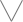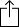C#
D#
E#
F#
G#
A#
B#
Chord
Scale
Custom
3nps
CAGED
None
Notes
Degrees
Intervals
None

1
2
3
4
5
6
7
8
9
10
11
12
13
14
15
16
17
18
19
20
21
22
23
24
E
B
G
D
A
E
F
F
G
G
A
B
B
C
C
D
D
E
F
F
G
G
A
B
B
C
C
D
D
E
C
C
D
D
E
F
F
G
G
A
B
B
C
C
D
D
E
F
F
G
G
A
B
B
G
A
B
B
C
C
D
D
E
F
F
G
G
A
B
B
C
C
D
D
E
F
F
G
D
E
F
F
G
G
A
B
B
C
C
D
D
E
F
F
G
G
A
B
B
C
C
D
B
B
C
C
D
D
E
F
F
G
G
A
B
B
C
C
D
D
E
F
F
G
G
A
F
F
G
G
A
B
B
C
C
D
D
E
F
F
G
G
A
B
B
C
C
D
D
E

G# Minor Pentatonic Scale for Guitar

G# Minor Pentatonic Scale is a pentatonic scale. Pentatonic scales have 5 notes, "penta" comes from the greek word "Pentateuch" which means 5.

The G# Minor Pentatonic Scale scale is derived from the G# Minor scale by taking scale degree 1, 3, 4, 5 and 7 of the scale.

G
B
C
D
F
Gm3
W
W
m3
W
Play scaleand then